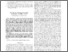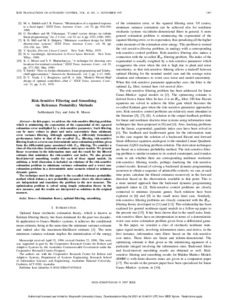# Risk-Sensitive Filtering and Smoothing via Reference Probability Methods

Dey, Subhrakanti and Moore, John B. (1997) Risk-Sensitive Filtering and Smoothing via Reference Probability Methods. IEEE Transactions on Automatic Control, 42 (11). pp. 1587-1591. ISSN 0018-9286Previewmore...Add this article to your Mendeley library

## Abstract

In this paper, we address the risk-sensitive filtering problem which is minimizing the expectation of the exponential of the squared estimation error multiplied by a risk-sensitive parameter. Such filtering can be more robust to plant and noise uncertainty than minimum error variance filtering. Although optimizing a differently formulated performance index to that of the so-called H1 filtering, risk-sensitive filtering leads to a worst case deterministic noise estimation problem given from the differential game associated with H1 filtering. We consider a class of discrete-time stochastic nonlinear state-space models. We present linear recursions in the information state and the result for the filtered estimate that minimizes the risk-sensitive cost index. We also present fixed-interval smoothing results for each of these signal models. In addition, a brief discussion is included on relations of the risk-sensitive estimation problem to minimum variance estimation and a worst case estimation problem in a deterministic noise scenario related to minimax dynamic games. The technique used in this paper is the so-called reference probability method which defines a new probability measure where the observations are independent and translates the problem to the new measure. The optimization problem is solved using simple estimation theory in the new measure, and the results are interpreted as solutions in the original measure.

Item Type: Article Estimation theory; optimal filtering; smoothing; Faculty of Science and Engineering > Electronic EngineeringFaculty of Science and Engineering > Research Institutes > Hamilton Institute 14404 https://doi.org/10.1109/9.649727 Subhrakanti Dey 10 May 2021 14:05 IEEE Transactions on Automatic Control IEEE YesItem control page